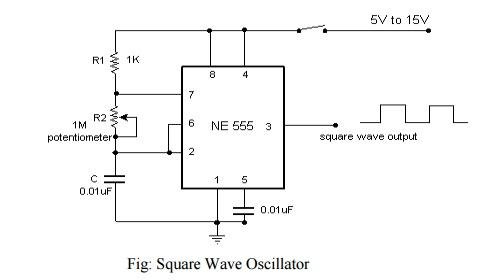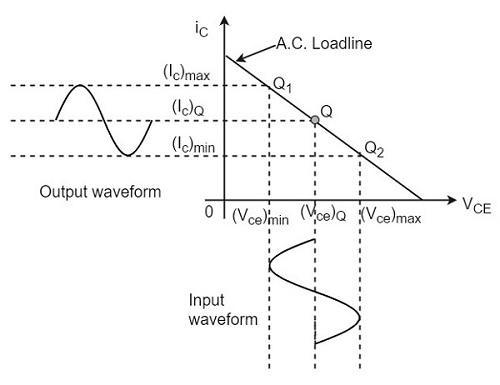# Analog Circuit Design Technical Interview Questions

### Que.15: What is Filter? Why filter is used?

Answer: A filter circuit is a device that converts the pulsating output of a rectifier into a steady-state dc level. It is essential to reduce the ripples from the pulsating dc supply available from rectifier circuits to the minimum to protect the load from damage. This is achieved by using a filter or smoothing circuit which removes the ac components and allows only dc components to reach the load. The filter circuit is always placed between the rectifier and load.

### Que. 90 What is the Schmitt trigger oscillator?

• An astable multivibrator is equivalent to Schmitt trigger with an additional RC network, hence called a Schmitt trigger oscillator.
• It is a circuit whose output does not have any stable state. Output has two quasi-stable states, and output keeps toggling between these two states without any external input. Hence it generates a square waveform. So this circuit is also known as a square waveform generator or free-running oscillator.
•### Que.71 What are the advantages of using a Class A  power amplifier? Where do we use the class A amplifier?

• Advantage of using a class A amplifier is that we keep the operating point at the center of the load line, hence the output is undistorted.
•• Class A amplifier is mostly used as a small-signal amplifier or voltage amplifier because it provides undistorted output. It is also used in high-frequency audio systems as an audio frequency power amplifier.
• ### Que.44 What is the early effect or base width modulation in BJT? What are its consequences?

• It refers to the decrease in effective base width due to a decrease in reverse bias voltage across the collector junction.
• The early effect has three consequences:
• Large signal current (alpha) increase- as Vcb increases effective base width decrease which implies a decrease in no. of recombination or increase in collector current, hence alpha increases.
• For small increases in alpha, beta(small-signal current gain) increases by a large amount.
• Emitter current Ie increases due to an increase in hole concentration gradient.
• ### Que.9: Explain the timer IC-55

Answer: IC-555 is a timer IC that is used in a stable multivibrator, square wave generator, triangular wave generator, pulse modulator, and pulse detector circuits.

### Que.1: What do you mean by operational Amplifier?

Answer: An operational amplifier is a direct-coupled, high gain amplifier used for some mathematical operations such as addition, subtraction, multiplication, integration, differentiation, logarithmic operations, etc.

### Que.55 What is a buffer circuit? How does BJT act as a buffer circuit?

• Buffer circuit: A circuit that is used for impedance matching between source and load circuits. So that maximum power is transferred from source to load circuit.
• A common-collector amplifier in BJT is used as a voltage buffer between source and load as it has large input resistance, smaller output resistance, and unity voltage gain.
•• The common base amplifier in BJT is used as a current buffer between source and load as it has smaller input resistance, large output resistance, and unity current gain.
• ### Que.6: Discuss the SVRR?

Answer: The full form of SVRR is the supply voltage rejection ratio. The change in an op-amp offset voltage caused by variation in supply voltage is called Supply voltage rejection ratio (SVRR).

### Que.16: What are the commonly used types of filter circuits?

Answer: 1. Series Inductor Filter 2. Shunt Capacitor Filter 3. Choke Input Filter 4. Capacitor input or Pie Filter